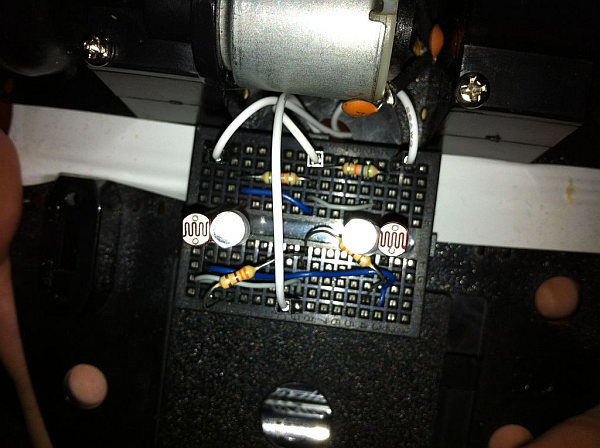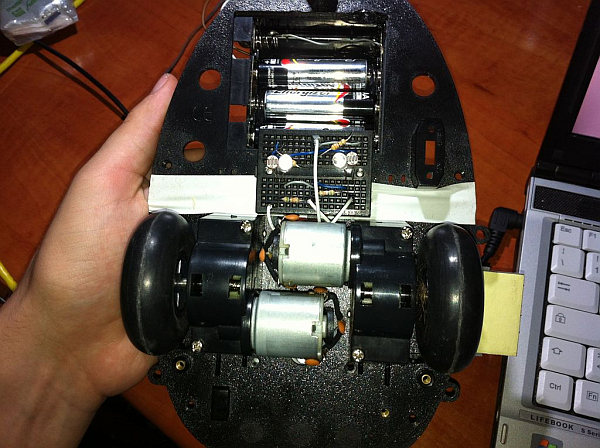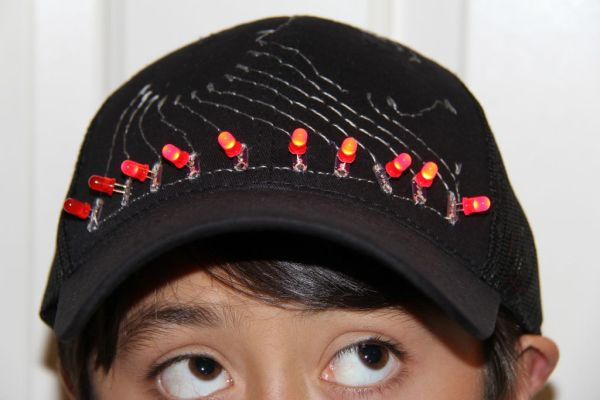# [Arduino] Linefollower with photoresistors

I built a linefollower robot that could be used in many different ways, and I summed up my project in some steps.
To begin with, all you need is the following parts:## Step 1: Robot frame

Parts List:
-Robot frame
-Two wheels
-Two DC motors

How to:
I used an old robot frame with two wheels commanded by two DC motors, but you can use an equivalent solution as well.

## Step 2: Sensor ir + photoresistorParts List:
-Two leds
-Two photoresistors
-Two 56k resistors
-Two 330 resistors
-Some wire

How to:
Calculate the distance between the two pairs of leds and photoresistors basing on the width of your tape.
Position the photoresistors and the leds as seen in the pictures.
Connect leds to two resistors of 330 ohm and put some non trasparent tape around it to prevent any interference with the photoresistors
Place the mini breadboard in the lower part of the frame.

## Step 3: Motor controller

Parts List:
-Some wire
-Diodes (if not already included into the motor controller)
-Motor controller
-Three 1.5v batteries

Sensors Assembling:
Plug in the input pins of bridge A on pins 11 and 12 of Arduino.
Plug in the input pins of bridge B on pins 6 and 5 of Arduino.
Connect with some diodes the output pins of each bridge to motors, if needed.
Connect the logic supply voltage (Vss) to 5v of Arduino and connect the ground of motor controller to the gnd of arduino.
Connect the supply voltage (Vs) to 3 AA batteries.

### Major Components in Project

-Robot frame
-Two wheels
-Two DC motors
-Two leds
-Two photoresistors
-Two 56k resistors
-Two 330 resistors
-Some wire
-Diodes (if not already included into the motor controller)
-Motor controller(i used L298)
-Three 1.5v battery
-Arduino UNO

For more detail: [Arduino] Linefollower with photoresistors